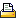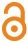nieuwe zoekopdracht
 [ meld een fout in dit record ] mandje (0): toevoegen | toonA theoretical basis for salinity intrusion in estuariesZhang, Z. (2019). A theoretical basis for salinity intrusion in estuaries. PhD Thesis. Delft University of Technology: Delft. xviii, 120 pp. https://hdl.handle.net/10.4233/uuid:d95a29ff-8865-4484-864a-74fd75028292Thesis info: Savenije, Hubert, promotor Wang, Zheng Bing, co-promotor

 Author keywords Alluvial estuary, salinity intrusion, empirical model, predictive equations, maximum power concept

 Auteur Top Zhang, Z.

 Abstract Saltwater intrusion is a crucial issue in estuaries. The spread of salinity is described by the dispersion coefficient. A purely empirical equation which links the effective tidal average dispersion to the freshwater discharge was developed by Van der Burgh . Combining it with the salt balance equation, Savenije  derived a one-dimensional model for salinity intrusion in estuaries. This Van der Burgh model has performed surprisingly well around the world. However, the physical basis of the empirical Van der Burgh coefficient (퐾) is still weak. This study provides a theoretical basis for the Van der Burgh method and presents alternative equations. MacCready  presented a theoretical expression for the dispersion coefficient following a reductionist approach. Comparing the density-related parts of the equations of the dispersion coefficient developed by Savenije and MacCready, a predictive equation is obtained for the coefficient 퐾 using physical parameters. In addition, a new box-model has been developed considering the longitudinal densitydriven gravitational circulation and the lateral tide-driven horizontal circulation. The coefficient 퐾 (closely related to the Van der Burgh’s coefficient) is used as an index of the density-driven mixing mechanism while the tide-driven part is included by assuming that it is proportional to the longitudinal dispersion. This model is validated in sixteen alluvial estuaries worldwide by using calibrated 퐾 values (and the boundary conditions). These calibrated values correspond well with the predicted values from the theoretical derivation, revealing that 퐾 has smaller values when the tide is stronger. From a system perspective, alluvial estuaries are free to adjust dissipation processes to the energy sources that drive them. The potential energy of the river flow drives mixing by gravitational circulation. The maximum power concept assumes that the mixing takes place at the maximum power limit. To describe the complex mixing processes in estuaries holistically, different assumptions had to be made. The maximum power concept did not work satisfactorily when estuaries were assumed as isolated systems. However, by including the accelerating moment provided by the freshwater discharge, the open estuary system could be solved in analogy with Kleidon  applying the maximum power concept. A new expression for the dispersion coefficient due to gravitational circulation has been derived and solved in combination with the advection-dispersion equation. This maximum power model works well in eighteen estuaries with a large convergence length, providing an alternative equation for the dispersion. These estuaries also have larger calibrated 퐾 values by the Van der Burgh method, revealing a relation between the empirical coefficient 퐾 and the geometry. All these models: the Van der Burgh model, the box-model, and the maximum power model, can describe the longitudinal salinity profiles. The comparison between these models implies that the empirical Van der Burgh coefficient is associated with the geometry and stratification conditions. Finally, new predictive equations have been obtained by regression with physical-based parameters which make the Van der Burgh salinity intrusion method predictive with a solid theoretical basis.

 Alle informatie in het Integrated Marine Information System (IMIS) valt onder het VLIZ Privacy beleid Top | Auteur
IMIS is ontwikkeld en wordt gehost door het VLIZ, voor meer informatie contacteer.